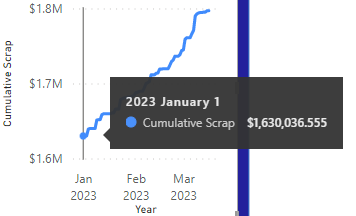cancel
Showing results for
Did you mean:Helper I

## Cumulative Scrap Cost - Only show this year

I am trying to show cumulative scrap cost for only this year but my data has multiple years worth of information in it. I'm very close - and but its starting at the amount that I started the year with. I'd like to show this same thing, but have January 1 start at 0. I think my cumulative scrap formula must need another and statement? But I can't seem to get it right.

Scrap Cost is the individual line items in my table, Scrap date is the day in that table that correlates to that cost, and calendar is just built out DAX Calendar

``````Cumulative Scrap =
CALCULATE(
SUM(DMR_Scrap[ScrapCost]), FILTER(ALLSELECTED(DMR_Scrap), DMR_Scrap[ScrapDate Only] <= Max('Calendar'[Date]) && Date(2023, 1,1) <= Max('Calendar'[Date])))``````Not sure this one is necessary to solve - but for reference!

``````Calendar =
VAR
_startDate = EDATE(Today(), -14)
VAR
_endDate = EDATE(TODAY(), 0)
RETURN
, "Year", Year([Date])
, "Year Month", YEAR([Date]) & " " & FORMAT([Date], "mmm")
, "Quarter", "Q" & QUARTER([Date])
, "Month Number", MONTH([Date])
, "Month", FORMAT([Date], "mmm")
, "Week", WEEKNUM([Date], 1)
, "Year - Week", Year([Date]) & " " & WEEKNUM([Date])
, "Day", Day([Date])
, "Date Slicer"
, IF([Date] = TODAY(), "Today"
, IF([Date] = TODAY() - 1, "Yesterday"
, FORMAT([Date], "mm/dd/yyyy") & ""
)
)
)``````Super User

@DemingPDCA Try:

``````Cumulative Scrap =
CALCULATE(
SUM(DMR_Scrap[ScrapCost]), FILTER(ALLSELECTED(DMR_Scrap), DMR_Scrap[ScrapDate Only] <= Max('Calendar'[Date]) && Date(2023, 1,1) <= Max('Calendar'[Date]))) + 0``````

Become an expert!: Enterprise DNA
External Tools: MSHGQM
Latest book!:
Mastering Power BI 2nd EditionDAX is easy, CALCULATE makes DAX hard...